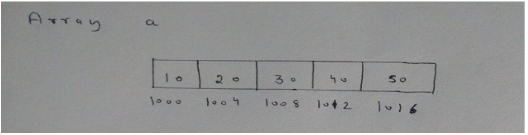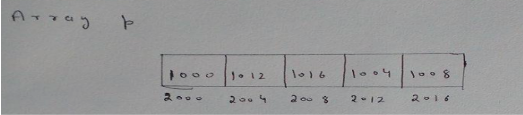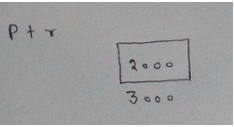# GATE | GATE-CS-2015 (Set 3) | Question 65

• Difficulty Level : Medium
• Last Updated : 18 Jan, 2018

Consider the following C program.

Attention reader! Don’t stop learning now.  Practice GATE exam well before the actual exam with the subject-wise and overall quizzes available in GATE Test Series Course.

Learn all GATE CS concepts with Free Live Classes on our youtube channel.

 `# include ``int` `main( )``{``  ``static` `int` `a[] = {10, 20, 30, 40, 50};``  ``static` `int` `*p[] = {a, a+3, a+4, a+1, a+2};``  ``int` `**ptr = p;``  ``ptr++;``  ``printf``(``"%d%d"``, ptr - p, **ptr};``}`

The output of the program is _________
(A) 140
(B) 120
(C) 100
(D) 40

Explanation:

In order to simplify programs involving complex operations on pointers, we suggest you to draw proper diagrams in order to avoid silly mistakes. Let’s assume that integer is of 4 Bytes and Pointer size is also 4 Bytes.
Let’s assume array a Base address is 1000. Array name actually holds the array base address.Let’s assume array p Base address is 2000.Double Pointer ptr Base Address is 3000.Now ptr is actually pointing to the first element of array p. ptr++ will make it point to the next element of array p. Its value will then change to 2004.
One of the Rule of Pointer Arithmetic is that When you subtract two pointers, as long as they point into the same array, the result is the number of elements separating them.
ptr is pointing to the second element and  p is pointing to the first element so ptr-p will be equal to 1(Excluding the element to which ptr is pointing).
Now ptr = 2004 —–> *(2004) = 1012 —-> *(1012) —-> 40.